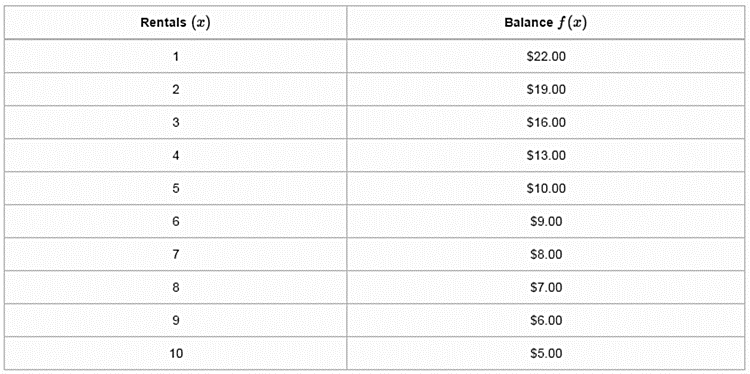Mathematics
Easy

Question

# Luke has a \$ 25 gift card to pay for video game rentals. Which piecewise-defined function best represents the balance remaining on the gift card, f(x) , after each game rental, x?## f(x) = 25 − 3x , 0 ≤ x ≤ 5f(x) = 15 − x , 6 ≤ x ≤ 10f(x) = 25 − 3x , 0 < x <5f(x) = 15 − x , 6 < x < 10f(x) = 25 + 3x , 0 ≤ x ≤ 5f(x) = 15 + x , 6 ≤ x ≤ 10f(x) = 25 + 3x , 0 < x < 5f(x) =15 + x , 6 < x < 10Hint:

## The correct answer is: f(x) = 25 − 3x , 0 ≤ x ≤ 5f(x) = 15 − x , 6 ≤ x ≤ 10

### x = 5, f(x) = 25 – 3x = 25 – 15 = 10x = 6, f(x) = 15 – 6 = 9Option A is the correct one.We are not taking the second option because it can be that he rents 0 games or he rents 5 games.So we have to include both of them.

Observe the problem carefully and look out for base cases.

### Related Questions to study#### With Turito Foundation.#### Get an Expert Advice From Turito.